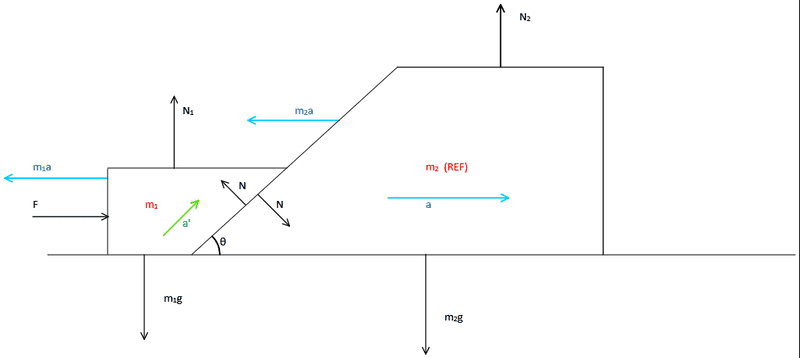# NLM Problem: More variables than equations

Seeker220
Homework Statement:
Two blocks initially at rest are kept in contact as shown in the figure. A force F is applied on the left block. The blocks are of masses ##m_1## and ##m_2##. Find the acceleration of the blocks. All surfaces are smooth.
Relevant Equations:
##Force = Mass \times Acceleration##Attempt at Solution::

##m_2## is chosen as reference frame and FBD is drawn as shown above. We get the following equations:

From ##m_1## by choosing axes along and perpendicular to acceleration of ##m_1## w.r.t ##m_2## (##a'##):

$$m_1 a' + m_1 a \cos{\theta} + m_1 g \sin{\theta} = N_1 \sin{\theta} + F \cos{\theta}$$
$$N+N_1 \cos{\theta} + m_1 a \sin{\theta} = m_1 g \cos{\theta} + F \sin{\theta}$$

From ##m_2## by choosing horizontal and vertical axes:
$$N_2 = N \cos{\theta} + m_2 g$$
$$m_2 a = N \sin{\theta}$$

Which gives us four equations, but five variables, namely ##a',a,N_1 ,N_2, N##

Homework Helper
Gold Member
Your FBD is incorrect. ##m_1a## and ##m_2a## are not forces. If you consider the two blocks together as your system, what is the net horizontal force acting on that system? What is its horizontal acceleration? Start from there then draw two separate FBDs for each block.

•topsquark, erobz and Seeker220
Gold Member
Please label the coordinates on the diagram, and the associated coordinate with each equation you have written down.

•topsquark and Seeker220
Seeker220
Your FBD is incorrect. ##m_1a## and ##m_2a## are not forces. If you consider the two blocks together as your system, what is the net horizontal force acting on that system? What is its horizontal acceleration? Start from there then draw two separate FBDs for each block.
umm.. ##m_1a## and ##m_2a## are pseudo forces because accelerating frame ##m_2## (with acceleration a towards right) is my Reference frame.

Net horizontal force on the two blocks as a system is F.
Sum of mass × horizontal acceleration of two blocks is F.

But how can you comment about their individual horizontal acceleration?

Homework Helper
Gold Member
2022 Award
Think about a' and N1. can both be nonzero?

•topsquark, erobz and Seeker220
Gold Member
Why ##N_1## participating in the sum of the forces if you assume the small mass is accelerating up the incline?

There are probably a few errors here. You should do as @kuruman suggest and present accurate FBD's of each block.

•topsquark and Seeker220
Seeker220
Think about a' and N1. can both be nonzero?
I think this actually solves it. Ok trying and editing to final solution...

Gold Member
So what did you get for ##a## and ##a'## as labeled in your diagram?

Staff Emeritus
•